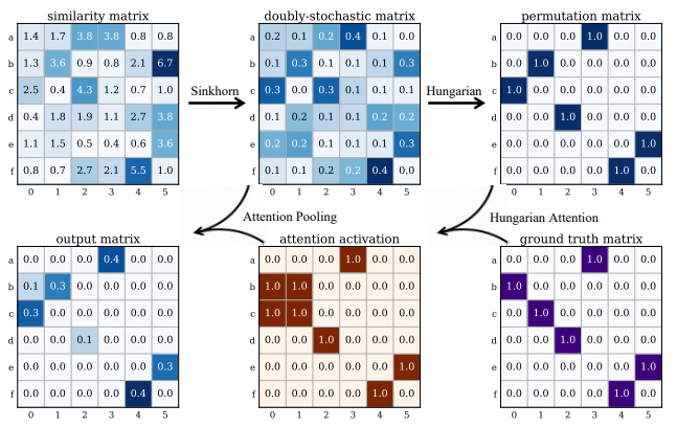# PermutationLossHung¶

class src.loss_func.PermutationLossHung[source]

Binary cross entropy loss between two permutations with Hungarian attention. The vanilla version without Hungarian attention is PermutationLoss.

$\begin{split}L_{hung} &=-\sum_{i\in\mathcal{V}_1,j\in\mathcal{V}_2}\mathbf{Z}_{ij}\left(\mathbf{X}^\text{gt}_{ij}\log \mathbf{S}_{ij}+\left(1-\mathbf{X}^{\text{gt}}_{ij}\right)\log\left(1-\mathbf{S}_{ij}\right)\right) \\ \mathbf{Z}&=\mathrm{OR}\left(\mathrm{Hungarian}(\mathbf{S}),\mathbf{X}^\text{gt}\right)\end{split}$

where $$\mathcal{V}_1, \mathcal{V}_2$$ are vertex sets for two graphs.

Hungarian attention highlights the entries where the model makes wrong decisions after the Hungarian step (which is the default discretization step during inference).

Note

For batched input, this loss function computes the averaged loss among all instances in the batch.

A working example for Hungarian attention:forward(pred_dsmat: torch.Tensor, gt_perm: torch.Tensor, src_ns: torch.Tensor, tgt_ns: torch.Tensor) torch.Tensor[source]
Parameters
• pred_dsmat$$(b\times n_1 \times n_2)$$ predicted doubly-stochastic matrix $$(\mathbf{S})$$

• gt_perm$$(b\times n_1 \times n_2)$$ ground truth permutation matrix $$(\mathbf{X}^{gt})$$

• src_ns$$(b)$$ number of exact pairs in the first graph (also known as source graph).

• tgt_ns$$(b)$$ number of exact pairs in the second graph (also known as target graph).

Returns

$$(1)$$ averaged permutation loss

Note

We support batched instances with different number of nodes, therefore src_ns and tgt_ns are required to specify the exact number of nodes of each instance in the batch.

training: bool